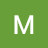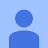8th Maths ICSE || Introduction to Sets || Maths Tutor || Rachna Sagar || Chapter-5 Sets ||8th Maths ICSE || Introduction to Sets || Maths Tutor || Rachna Sagar || Chapter-5 Sets ||

8th Maths ICSE || Introduction to Sets || Maths Tutor || Rachna sagar !! For the 8th maths icse Link of chapter 12 Expansions from the book by Rachna Sagar Magic of mathematics by maths tutor is given below

algebra tutors,Class 8 Maths icse,Class 8 Maths icse introduction of Sets,icse 8th math chapter 5 Sets,8th class icse math by Rachna Sagar,Magic of mathematics,Chapter-5 Sets introduction,8th math ch-5 Sets introduction,Class 8 icse math chapter 5 Sets intro,Maths Tutor,Easy explanation,Introduction to Sets class 8 icse math,icse 8th maths,icse 8th maths sets,Rachna Sagar,Introduction to Sets,8th maths icse

algebratutors.org

3 Responses to “8th Maths ICSE || Introduction to Sets || Maths Tutor || Rachna Sagar || Chapter-5 Sets ||”

1.Micky Uppal says:

Keep it up sir👍

2.Raman Kakkar says:

Well done sir

3.Kashish X says:

good👍